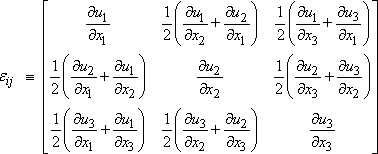Streamlines from strain rate tensor

I was reading about strain rate tensors and other kinematic properties of fluids that can be obtained if we know the velocity field V = (u, v, w). It got me wondering if I can sketch streamlines if I have the strain rate tensor with me to start with. Let's say I have the strain rate tensor:Would it now be possible to sketch the flow field and determine the flow direction from this? If yes, how?

Also, in 2D potential flows streamlines are perpendicular to potential lines. Does this mean in 3D the set of streamlines will be a plane with a line perpendicular to it? And can this also be explained with just the help of the strain rate tensor above by solving an eigenvalue problem?

Orodruin
Staff Emeritus
Homework Helper
Gold Member
2021 Award
No. The strain rate tensor can be zero while maintaining a steady flow. Think a body of fluid in uniform translation and rotation.

Also, in 2D potential flows streamlines are perpendicular to potential lines. Does this mean in 3D the set of streamlines will be a plane with a line perpendicular to it?

For a potential flow ##\vec v = \nabla \phi## for some ##\phi##. The gradient is always orthogonal to the level surfaces of a scalar function. The streamlines for an irrotational flow will therefore always be perpendicular to the potential surfaces.

Chestermiller
Mentor
I was reading about strain rate tensors and other kinematic properties of fluids that can be obtained if we know the velocity field V = (u, v, w). It got me wondering if I can sketch streamlines if I have the strain rate tensor with me to start with. Let's say I have the strain rate tensor:

View attachment 204609

Would it now be possible to sketch the flow field and determine the flow direction from this? If yes, how?

Also, in 2D potential flows streamlines are perpendicular to potential lines. Does this mean in 3D the set of streamlines will be a plane with a line perpendicular to it? And can this also be explained with just the help of the strain rate tensor above by solving an eigenvalue problem?
No. Aside from not knowing the velocity vector at any given location (which would provide the constant of integration), the strain rate tensor represents only the symmetric part of the velocity gradient tensor, and does not include the antisymmetric part (i.e., the vorticity tensor) which describes rotation of the fluid parcels.